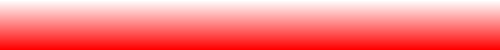# Lorentz attractorMove with the mouse over the table

 One dimensional maps can help to understand ordinary differential equations:: If you cut the Lorentz attractor at a suitable place with a plane, the intersection points form a pointset lies approximately on an interval. This means that if we look at a solution curve which starts on a point of that interval, the next time this curve will intersect the plane will again be on that interval. This return map is essentially a map on the interval. Indeed, when changing the parameters of the differential equation one finds bifurcation diagrams for periodic flow lines similar to the bifurcation diagram for the logistic map.

Back to the Math118r page